# differential Opamp Current measurement for low side & high side sensing - help needed

#### mishra87

Joined Jan 17, 2016
958
Hi Guys,

Can any explain the concept for below circuit . What is the impact of this measurement
Is my equation is correct to find Vout in both cases ?

Note : This is not university question. This is the practical application for current measurement of two different device using single op amp.

Case 1 :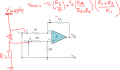Case 2 : What is equation for this case ?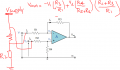Last edited:

#### SEhsan_Analog

Joined Aug 8, 2020
2
Is that an ideal OP-amp?
In other words, is 'A' a bounded amount?
Or it's equal to infinity?

#### Alec_t

Joined Sep 17, 2013
12,807
For many practical opamps the inputs are not rail-to-rail, so you would need to consult the datasheet of the op-amp you intend to use to check if it is capable of accepting the high-side or low-side input voltage as a valid input.

#### mishra87

Joined Jan 17, 2016
958
For many practical opamps the inputs are not rail-to-rail, so you would need to consult the datasheet of the op-amp you intend to use to check if it is capable of accepting the high-side or low-side input voltage as a valid input.

My intention was to know the difference in output equation for both cases described above.
Do we use same equation to get Vout ?

Regards

#### AlbertHall

Joined Jun 4, 2014
12,020
Is Vsupply the same polarity in both cases?
If so why are the connections to the shunt reversed?

#### mishra87

Joined Jan 17, 2016
958
Is Vsupply the same polarity in both cases?
If so why are the connections to the shunt reversed?
There is two source.

1st schematic - battery charging path
2nd schematic - Battery discharging path

So objective is to measure charging and discharging current of battery. Schematic is working working fine only i wanted to understand Vout calculation for both cases

#### mishra87

Joined Jan 17, 2016
958
Hello Guys,

Did anyone get a chance to have a look on above differential measurement ?

Regards

#### Alec_t

Joined Sep 17, 2013
12,807
Do we use same equation to get Vout ?
Yes. Both measurements are high-side, with just V1 and V2 swapped over. The equation is correct by my reckoning.

#### mishra87

Joined Jan 17, 2016
958
Yes. Both measurements are high-side, with just V1 and V2 swapped over. The equation is correct by my reckoning.
Many thanks for confirmation !

#### mishra87

Joined Jan 17, 2016
958
Hello Guys,

I have 8.4V battery source and i want to measure the load current using differential amplifier as per below. It's design constraint to measure current high at high side.

Precondition : I have 4V opamp supply voltage and microcontroller supply is 4V so i think no discussion for supply voltage.
Resistance R1, R2, R3 and R4 are not same. Opamp is MCP6001UT
https://www.mouser.in/datasheet/2/268/21733j-740845.pdf

1. Can i measure the current for below circuit, if no what is the reason ?
2. What is common mode voltage in datasheet, does Inverting and non inverting terminal of opamp will get damage ?
3. Is there any other method to measure current for this application?
4. Calculation for opamp output voltage ?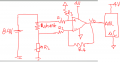#### DickCappels

Joined Aug 21, 2008
8,705
1. Can i measure the current for below circuit, if no what is the reason ?
Yes. No problem.

2. What is common mode voltage in datasheet, does Inverting and non
inverting terminal of opamp will get damage ?

Common mode voltage is the voltage that appears on both the inverting and non-inverting inputs. In normal opration with negative feedback these two voltages are the same. #1 do not exceed datasheet limits and #2 in the case of the common mode voltage range operating outside this range but between the power supply rails will cause some opamps to invert their operation - in otherwords what was negative feedback becomes positive and the output locks up. Usually not a desirable situation. Other undesirable operation sycg as clipping of the input signals may also result.

3. Is there any other method to measure current for this application?
Stuff to consider: Fluxgate magnetometer, hall effect current sensor, and commerically made isolation amplifiers, and flying capacitor commutation comes to mind.

4. Calculation for opamp output voltage ?
(IR drop of the shunt) x (Differential gain of the amplifier) + errors.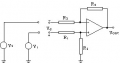Differential Mode and Common Mode Gain of Differential Amplifier. Gain of an amplifier is defined as VOUT/VIN. For the special case of a differential amplifier, the input VIN is the difference between its two input terminals, which is equal to (V1-V2) as shown in the following diagram. Gain = VOUT/(V1-V2)

#### Alec_t

Joined Sep 17, 2013
12,807
1. Can i measure the current for below circuit, if no what is the reason ?
No. 8.4V on the input exceeds the maximum common mode value allowed (4.3V in your setup). You would have to use potential dividers on both the inverting and non-inverting inputs.

•OBW0549

#### mishra87

Joined Jan 17, 2016
958
1. Can i measure the current for below circuit, if no what is the reason ?
Yes. No problem.

2. What is common mode voltage in datasheet, does Inverting and non
inverting terminal of opamp will get damage ?

Common mode voltage is the voltage that appears on both the inverting and non-inverting inputs. In normal opration with negative feedback these two voltages are the same. #1 do not exceed datasheet limits and #2 in the case of the common mode voltage range operating outside this range but between the power supply rails will cause some opamps to invert their operation - in otherwords what was negative feedback becomes positive and the output locks up. Usually not a desirable situation. Other undesirable operation sycg as clipping of the input signals may also result.

3. Is there any other method to measure current for this application?
Stuff to consider: Fluxgate magnetometer, hall effect current sensor, and commerically made isolation amplifiers, and flying capacitor commutation comes to mind.

4. Calculation for opamp output voltage ?
(IR drop of the shunt) x (Differential gain of the amplifier) + errors.

View attachment 214886

Differential Mode and Common Mode Gain of Differential Amplifier. Gain of an amplifier is defined as VOUT/VIN. For the special case of a differential amplifier, the input VIN is the difference between its two input terminals, which is equal to (V1-V2) as shown in the following diagram. Gain = VOUT/(V1-V2)
Thanks you so much for valuable time !
Now i got some technical aspect you mentioned here. Lets us consider a examples.
Rshunt = 5mOhm
So Vshunt =5mOhm*10A =50mV

Opamp : R1 = 5K, R3 = 390K, R2=1K, R4=56K
So Gain = R4/R2 = 56K/1K = 56 ( i am not sure here what is the role of R1 & R3)
Vout = 50mV*56 = 2.8V (As per my calculation opamp Vout = 2.8V)

Now i am confused here.
Since Vs = 8.4V, V+(Non Inverting) = 8.4, V-(Inverting) = 8.35V, Opamp Vcc = 4V, Vss =0
As per MCP6001 datasheet : VCMR = Vss-0.3V to Vdd+0.3 = -0.3 to 4.3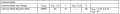Now V+ = 8.4 and V- = 8.35V which is greator than -0.3 to 4.3 so will this damage the opamp inverting & non inverting pin since Vss =0V and Vdd=4V of an opamp...?

This is doubt basically i have in my mind so could anyone one help me out here ?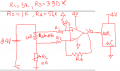Last edited:

#### mishra87

Joined Jan 17, 2016
958
No. 8.4V on the input exceeds the maximum common mode value allowed (4.3V in your setup). You would have to use potential dividers on both the inverting and non-inverting inputs.
Post #11 says you can measure but you say i can not measure.
I made calculation in post #13 so could you please check and suggest ?
How can i calculate VCM for above opamp and values ?

Last edited:

#### OBW0549

Joined Mar 2, 2015
3,566
Post #11 says you can measure but you say i can not measure.
@Alec_t is correct in post #12, for exactly the reasons he gives: for an MCP6001 operated off 4 volts, you would be exceeding its input common mode range. Your circuit won't work.

I made calculation in post #13 so could you please check and suggest ?
If you want a correct differential measurement of the voltage across the shunt resistor, the ratio R3/R1 absolutely MUST be equal to the ratio R4/R2. Otherwise you will not be making a true differential measurement.

But first, you need to fix the common mode voltage problem. Until you do that, there's no point in going any further.

Suggestion: instead of an MCP6001, try using an LT1490A; this op amp (actually a dual op amp) has an unusual characteristic in that its input common mode range actually exceeds the supply voltage, and in fact the inputs can go up as high as +44 volts regardless of the supply.

Change to an LT1490A, and then get on with equalizing the R3/R1 and R4/R2 ratios.

#### Alec_t

Joined Sep 17, 2013
12,807
Last edited:
•DickCappels

#### mishra87

Joined Jan 17, 2016
958
Thanks for valuable time !

Here is some query from my side in your design.

1. Sinnce you connected opamp supply to 8.4V so there should not be any issue with VCM so we should not worry about VCM here. In my application opamp supply was 4V as you may see again.

2. In your circuit, Vcc of opamp is 8.4V and microcontroller power supply is 4V so there is chances that microcontroller will get damage because if opamp component fails there is a chance that uC ADC will see 8.4V at its pin which is above the its supply.

3. How did you calculate the gain of your circuit ?

4. What is use of additional resistance R4 ?

Regards,

#### mishra87

Joined Jan 17, 2016
958
@Alec_t is correct in post #12, for exactly the reasons he gives: for an MCP6001 operated off 4 volts, you would be exceeding its input common mode range. Your circuit won't work.

If you want a correct differential measurement of the voltage across the shunt resistor, the ratio R3/R1 absolutely MUST be equal to the ratio R4/R2. Otherwise you will not be making a true differential measurement.

But first, you need to fix the common mode voltage problem. Until you do that, there's no point in going any further.

Suggestion: instead of an MCP6001, try using an LT1490A; this op amp (actually a dual op amp) has an unusual characteristic in that its input common mode range actually exceeds the supply voltage, and in fact the inputs can go up as high as +44 volts regardless of the supply.

Change to an LT1490A, and then get on with equalizing the R3/R1 and R4/R2 ratios.
Thanks for you time !
LT1490A is to costly opamp to use application.
I found some current sensor amplifier which is cheaper and built in balancing resistor so i can select the gain. For this application 50 Gain would be enough. There in no issue of common mode voltage as it has wide range of VCM.

#### Alec_t

Joined Sep 17, 2013
12,807
1. Sinnce you connected opamp supply to 8.4V so there should not be any issue with VCM so we should not worry about VCM here.
Correct.
2. In your circuit, Vcc of opamp is 8.4V and microcontroller power supply is 4V so there is chances that microcontroller will get damage because if opamp component fails there is a chance that uC ADC will see 8.4V at its pin which is above the its supply.
Whatever circuit you use, if you're trying to measure something withe a high-side voltage of 8.4V then some component failure could fry a micro/ADC with a 4V supply.
3. How did you calculate the gain of your circuit ?
I didn't. I just tried various values for R5 to give a sensible output voltage in the sim.
4. What is use of additional resistance R4 ?
With R2 it forms a voltage divider, to bring the input voltage within the range that the opamp can handle. The division ratio has to be the same as R1/R3.

#### mishra87

Joined Jan 17, 2016
958
Now i am much aware of current measurement section.

I do not want new thread so i will be continue this thread to have better understanding in below topics although it is deep learning curve for me.

1. I want to simulate battery charging circuit as per below schematic but i am not sure if this circuit works. so i have two path i.e. battery charging and battery discharging. Battery charging path will be as per below.

So my question here is , will PMOS M1 & M2 will work ? if yes what the theoretical analysis ?
One more i want to add here is due to design constraint i have to use two mosfet in series.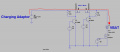2. battery discharging path will be as per below. Charging will not be done during battery discharging. So i have one charging and one discharging path.

So my question here, will PMOS M1 & M2 get turned ON during charging ? if yes how in terms of theory ?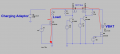Could anyone clarify my doubts here ?
Thanks once again !

#### Attachments

• 2.5 KB Views: 0